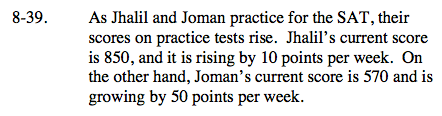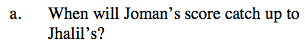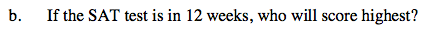### Home > CAAC > Chapter 8 > Lesson 8.1.4 > Problem8-39

8-39.
1.As Jhalil and Joman practice for the SAT, their scores on practice tests rise. Jhalil's current score is 850, and it is rising by 10 points per week. On the other hand, Joman's current score is 570 and is growing by 50 points per week. Homework Help ✎

1. When will Joman's score catch up to Jhalil's?

2. If the SAT test is in 12 weeks, who will score highest?Write two equations representing their scores.
Jhalil: y = 10x + 850 x = time (weeks)
Joman: y = 50x + 570 y = score (points)

Set the two equations equal to each other and solve for x.Substitute 12 for x in both the equations and compare the outcomes.